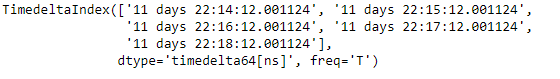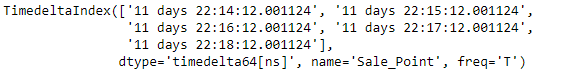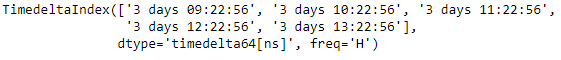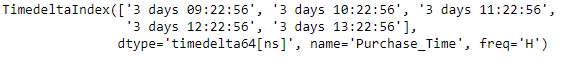Related Articles
Python | Pandas TimedeltaIndex.set_names
• Last Updated : 06 Jan, 2019

Python is a great language for doing data analysis, primarily because of the fantastic ecosystem of data-centric python packages. Pandas is one of those packages and makes importing and analyzing data much easier.

Pandas` TimedeltaIndex.set_names()` function is used to set new names on the given TimedeltaIndex object. The function defaults to returning new index object.

Syntax : TimedeltaIndex.set_names(names, level=None, inplace=False)

Parameters :
names : name(s) to set
level : If the index is a MultiIndex (hierarchical), level(s) to set (None for all levels). Otherwise level must be None
inplace : if True, mutates in place

Return : new index (of same type and class…etc) [if inplace, returns None]

Example #1: Use `TimedeltaIndex.set_names()` function to set the names of the given TimedeltaIndex object.

 `# importing pandas as pd``import` `pandas as pd`` ` `# Create the TimedeltaIndex object``tidx ``=` `pd.TimedeltaIndex(start ``=``'11 days 22:14:12.001124'``, periods ``=` `5``, freq ``=``'T'``)`` ` `# Print the TimedeltaIndex object``print``(tidx)`

Output :Now we will use the `TimedeltaIndex.set_names()` function to set the name of the given TimedeltaIndex object.

 `# set the name of the object``tidx.set_names(names ``=``'Sale_Point'``)`

Output :As we can see in the output, the `TimedeltaIndex.set_names()` function has returned a new object and it has also set the name of the returned object.

Example #2: Use `TimedeltaIndex.set_names()` function to set the names of the given TimedeltaIndex object.

 `# importing pandas as pd``import` `pandas as pd`` ` `# Create the TimedeltaIndex object``tidx ``=` `pd.TimedeltaIndex(start ``=``'03 days 09:22:56'``, periods ``=` `5``, freq ``=``'H'``)`` ` `# Print the TimedeltaIndex object``print``(tidx)`

Output :Now we will use the `TimedeltaIndex.set_names()` function to set the name of the given TimedeltaIndex object.

 `# set the name of the object``tidx.set_names(names ``=``'Purchase_Time'``)`

Output :As we can see in the output, the `TimedeltaIndex.set_names()` function has returned a new object and it has also set the name of the returned object.

Attention geek! Strengthen your foundations with the Python Programming Foundation Course and learn the basics.

To begin with, your interview preparations Enhance your Data Structures concepts with the Python DS Course.

My Personal Notes arrow_drop_up